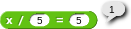# Snap! Sr. (In progress, brainstorming topic/sharing current)

Spin off from Snap! Jr., Snap! Sr. (senior) is really a library of mathematical functions, and other stuff. There is also just some cool blocks I made. This could expand in the future but I have programmers block and need ideas.

Mmmm-Not so sure this is correct... x should equal 25, not 1.

(For earthrulerr in case he doesn't understand)

The multiplicative inverse of division is multiplication. Also division isn't commutative.

So x/1 isn't the same as 1/x. If x=0 then 0/1 equals 0 but 1/0 equals undefined.

So in the case x should be 25 to get 5. You should report ans*secondnum

Fixed*

Thank you sir.

The num +- x = eq and  num $nl pow blocks need to be fixed. How so? The definition of num +- x = eq is identical to x +- num = eq, so the subtraction will not work.  num$nl pow does not calculate the power of a number.

Fixed.

Yes….? One is unnecessary and is just for looks I guess, they are the same.

How so?

a + b = b + a
a - b ≠ b - a